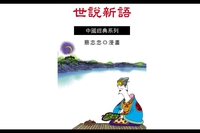世說新語

• 點閱：55
• 作者：
• 出版年：2007[民96]
• 出版社：蔡志忠
• 出版地：臺北市
• 集叢名：中國漫畫經典-中國經典系列章節

• 席不暇暖(p.1)
• 舍命全交(p.7)
• 割席絕交(p.14)
• 救人救到底(p.21)
• 喜怒不寄於顏(p.30)
• 庾公不賣馬(p.35)
• 阮裕焚車(p.41)
• 褚裒備四時之氣(p.45)
• 謝安教子(p.50)
• 以眼比月(p.55)
• 床頭盜酒飲(p.59)
• 鄧艾口吃(p.64)
• 楚囚對泣(p.68)
• 以簡對煩(p.73)
• 如來背痛(p.79)
• 如遊蓬戶(p.83)
• 廉者不求 貪者不與(p.87)
• 支道林愛馬(p.91)
• 文王之囿(p.96)
• 人言我憒憒 後人當思此憒憒(p.101)
• 鄭玄拜師(p.106)
• 向郭二莊(p.118)
• 白馬論(p.124)
• 官本臭腐，財本糞土(p.129)
• 三日不讀經 其言也無味(p.133)
• 七步成詩(p.137)
• 婦人之美 非誄不顯(p.142)
• 對子罵父是無禮(p.146)
• 阮修論鬼神(p.152)
• 義無虛讓(p.157)
• 臨刑不變色(p.163)
• 王戎不摘李(p.169)
• 東床快婿(p.176)
• 中夜奏胡笳(p.182)
• 謝安下棋如故(p.189)
• 劉備善乘邊守險(p.195)
• 見機而退(p.199)
• 王述晚成(p.207)
• 人所應無已不必無(p.213)
• 以長勝人 處長亦勝人(p.219)
• 王右軍論名士(p.222)
• 知我勝我自知(p.227)
• 不能言而能不言(p.231)
• 王大自是三月柳(p.236)
• 駑牛勝駑馬(p.241)
• 諸葛三兄弟(p.246)
• 阮裕兼有四人之美(p.251)
• 二流中人耳(p.257)
• 辭勝理不如(p.263)
• 卿喜傳人語不能複語卿(p.269)
• 吉人之辭寡(p.274)
• 武帝不忘哺乳恩(p.281)
• 錦被風波(p.287)
• 元帝戒酒(p.294)
• 闊(p.301)
• 合字玄機(p.307)
• 絕妙好辭(p.313)
• 何晏明惠若神(p.323)
• 日近日遠(p.329)
• 心靜自然涼(p.336)
• 王敦擊鼓(p.341)
• 王敦驅婢妾(p.348)
• 床頭捉刀人(p.353)
• 人小心不小(p.359)
• 周處除三害(p.364)
• 戴淵投劍(p.386)
• 蘭亭集序(p.392)
• 王仲宣好驢鳴(p.397)
• 為卿作驢鳴(p.402)
• 靈床鼓琴(p.409)
• 琴隨人亡(p.415)
• 觀點不同(p.423)
• 範宣不入公門(p.428)
• 許掾好遊山水(p.432)
• 慎勿為好(p.437)
• 王湛選妻(p.441)
• 韓母隱古幾(p.447)
• 委罪於木(p.452)
• 坐隱與手談(p.458)
• 畫人點睛(p.461)
• 顧愷之的畫論(p.466)
• 我成輕薄京兆尹(p.469)
• 竹林七賢(p.474)
• 劉伶戒酒(p.478)
• 劉昶的酒品(p.486)
• 房子是褲子(p.491)
• 張翰不留身後名(p.497)
• 拍浮酒池中(p.501)
• 萬裏長江哪能不彎(p.506)
• 不作送書郵(p.511)
• 不可一日無此君(p.515)
• 乘興而行興盡而返(p.520)
• 桓伊吹笛(p.526)
• 阮籍胸中有壘塊(p.533)
• 酒(p.537)
• 鳳(p.543)
• 無勳受祿(p.549)
• 山高水深(p.555)
• 狗洞自有狗出入(p.559)
• 我曬腹中書(p.564)
• 倒吃甘蔗(p.570)
• 白頸烏(p.575)
• 望梅止渴(p.580)
• 假戲真做(p.587)
• 曹操的詭計(p.599)
• 肝腸寸斷(p.605)
• 咄咄怪事(p.611)
• 王戎儉吝(p.615)
• 王戎有好李(p.620)
• 王戎重錢輕女(p.624)
• 美人行酒(p.629)
• 人乳養豬(p.636)
• 魏武殺妓(p.641)
• 天下要物惟《戰國策》(p.647)
• 賴其末不識其本(p.653)
• 蟻動入牛鬥(p.658)
• 荀粲情深(p.663)
• 德不足稱以色為主(p.670)
• 骨肉相圖(p.675)